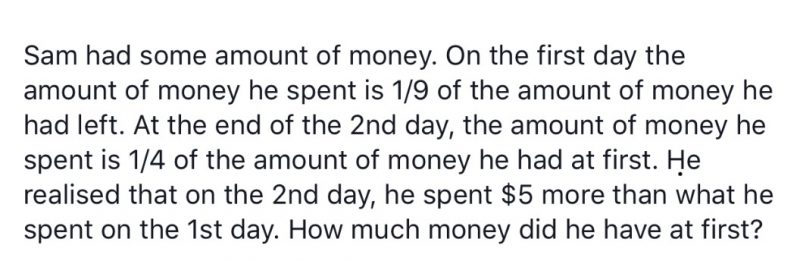# QuestionHi can help to solve this question. Thks

this is a badly-worded question due to the usage of the phrase “at first”.
if taken to mean the amount of money he had at the beginning of the question,
it would lead to an unreasonable answer of \$33.33~.

if you take it to mean the amount of money he had at the beginning of the
2nd day, you’ll get \$40 for the answer.

\$40 – \$4 = \$36 (4/36 -> 1/9)
1/4 of 36 = \$9
9-4 = \$5 (difference between the 2 day’s expenditure)

in any case, you should clarify the question with the setter.

0 Replies 0 Likes

Let the amount of money Sam had at first be 20 units
Amount of money spent on :
1st day : 2 units
2nd day : 5 units – 2 units = 3 units
3 units – 2 units = 1 unit = 5
20 units = 20 x 5 = 100

Ans : \$100.

0 Replies 0 Likes

This is a part whole question.

1.  Day 1 spend 1u. left 9u. Total 10u

2.  Day 2. 1/4 × 10 = 2.5u spend.

 Day 1 Day 2 left total Quantity 1u 2.5u 5.5u 9u

2.5u – 1u = 1.5u

1.5u = 5

1u = 3 1/3

9u = 9 × 3 1/3 = 30

Note

The question is misleading by the words “he spent 1/9 of what he had left”. Instead the word left should be ignored.

😊

0 Replies 0 Likes